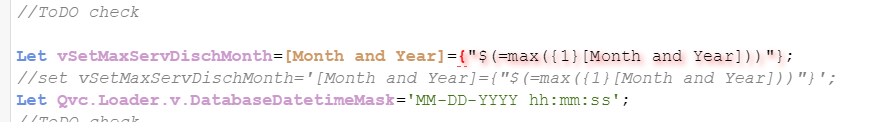# New to Qlik Sense

If you’re new to Qlik Sense, start with this Discussion Board and get up-to-speed quickly.

Announcements
Become an analytics expert with Qlik's new 15 week course: Applied Data Analytics using Qlik Sense. READ MORE
cancel
Showing results for
Did you mean:Partner

## Create Variable using Script and use it in the expression

Hello All,

I'm trying to create a variable using Load Script editor, and use that value in expressions.

I'm getting the syntax error for this,

Let vSetMaxServDischMonth=[Month and Year]={"\$(=max({1}[Month and Year]))"};

But when I create the same expression using expression editor and creating the variable and adding the expression, works fine.

ThanksLabels (4)

• ### Qlik Chart Functions

1 Solution

Accepted SolutionsLuminary Alumni

You are trying to create a literal value so you should use SET instead of LET

Set vSetMaxServDischMonth=[Month and Year]={"\$(=max({1}[Month and Year]))"};

If you wanted to create a literal with Let the string should be quoted as:

Let vSetMaxServDischMonth='[Month and Year]={"\$(=max({1}[Month and Year]))"}';

However, you will run into problems as the "\$(" will be interpreted in the script. So you must use Let with some kind of escaping of the \$( like:

Let vSetMaxServDischMonth=replace('[Month and Year]={"@(=max({1}[Month and Year]))"}', '@', '\$');

-Rob

3 RepliesPartner
aggregation like you have defined doesnot work in the script.
if you absolutly have to do it in script and not in variable section. you should do something like below

temp-table:
select Max([Month and year]) as MaxMonthYear resident YourTable;
let vMaxMonthYear = peek('MaxMonthYear');
drop table temp-table;Luminary Alumni

You are trying to create a literal value so you should use SET instead of LET

Set vSetMaxServDischMonth=[Month and Year]={"\$(=max({1}[Month and Year]))"};

If you wanted to create a literal with Let the string should be quoted as:

Let vSetMaxServDischMonth='[Month and Year]={"\$(=max({1}[Month and Year]))"}';

However, you will run into problems as the "\$(" will be interpreted in the script. So you must use Let with some kind of escaping of the \$( like:

Let vSetMaxServDischMonth=replace('[Month and Year]={"@(=max({1}[Month and Year]))"}', '@', '\$');

-RobPartner
Author

Thank you 🙂